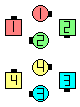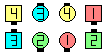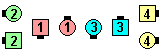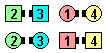Definitions of Square Dance Calls and Concepts
Explode The Diamond [C3B]Index -->  Plus  |  A1  |  A2  |  C1  |  C2  |  C3A  |  C3B  |  C4  |  NOL  |
Definitions (Text Only) -->  Plus  |  A1  |  A2  |  C1  |  C2  |  C3A  |  C3B  |  C4  |  NOL  |
 Find call:

 \$B8@8l(B2 \$B\$D\$N(B Diamond, 3/4 Tag, \$B\$^\$?\$OB>\$NE,@Z\$J(B formation \$B\$+\$i(B.

Centers \$B\$O(B Explode The Wave \$B\$r(B, Ends \$B\$O(B Circulate \$B\$r\$7(B Lead End U-Turn Back \$B\$r\$7\$^\$9(B.

Diamond \$B\$+\$i\$OJ?9T\$J(B Inverted Line \$B\$G=*\$o\$j\$^\$9(B.Explode The Diamond\$B\$NA0(B \$B8e(BExplode The Diamond\$B\$NA0(B \$B8e(BChoreography for Explode The DiamondComments? Questions? Suggestions?